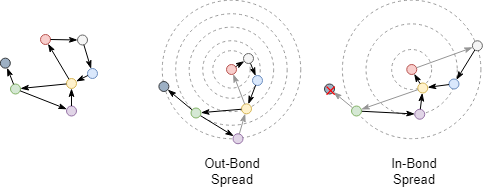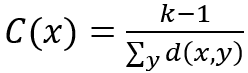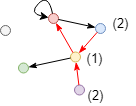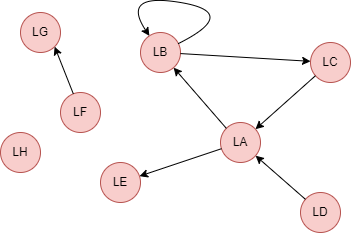# Change Nickname

Current Nickname:

Certifications

Certificate Issued at Valid until Serial No. File
Serial No. Valid until

Not having one? Apply now! >>>

Invoice

ProductName CreateTime ID Price File
ProductName CreateTime ID Price File

# Closeness Centrality

## Overview

Closeness centrality is used to measure the average shortest distance from node to other nodes in its connected component. This concept helps to select the closest nodes to each node in the connected component, so it's widely used in application scenarios such as the discovery of key social nodes and the location of functional places.

The range of values of closeness centrality is [0,1]; the larger the value, the closer the node is to the center.

Closeness centrality has various definitions in practical applications, it was originally proposed by Alex Bavelas in 1950.

Related material of this algorithm:

## Basic Concept

### Shortest Distance

The shortest distance is the number of edges in the shortest path between two nodes. The shortest path is searched by the BFS principle, if one node is regarded as the start node and another node is one of the K neighbors of the start node, value of K is the shortest distance from the start node to its K neighbors. Please read the chapter K-Hop Whole Graph for the introduction to BFS and K neighbors.Examine the shortest distance between the red and green nodes in the undirected graph above. Since it's an undirected graph, whether using the red or green node as the start node, they are both 2-Hop neighbors of each other, that is, the shortest distance between the two nodes is 2.Examine the shortest distance between the red and green nodes again after modifying the undirected graph to directed graph, the direction of edges in the path should be considered now. Outbound shortest distance from the red node to the green node is 4, inbound shortest distance to the red node from the green node is 3.

### Closeness Centrality

Closeness centrality calculated by this algorithm is derived from the original concept, it's defined as the reciprocal of the average shortest distance from a node to the other nodes that's connected.where `x` is the start node to be calculated, `y` is any node that connects with `x` (along the outbound edges, inbound edges or edges with direction ignored; `x` is excluded), `d(x,y)` is the shortest distance from `x` to `y`, `k-1` is the number of `y`.Calculate the inbound closeness centrality of the red node in the graph above. It can connect to the blue, yellow and purple three nodes, so the closeness centrality is `3 / (2 + 1 + 2) = 0.6`. Please note that the green and grey nodes are not reachable in the inbound direction, so they are not included in the calculation.

Closeness Centrality algorithm consumes considerable computing resources because all the shortest paths from a node in the whole graph are to be calculated. You may perform sampling closeness centrality calculation on GraphSet with more than 10,000 nodes, the suggested number of samples is the logarithm based on 10 `log(number of nodes)`; user can configure whether to sample or not.

## Special Case

### Lonely Node, Disconnected Graph

Lonely node is not connected with any other node, so its closeness centrality is 0. Lonely node doesn't participate in any closeness centrality calculation.

Nodes in one connected component must NOT participate in the closeness centrality calculation of nodes in other connected components. For disconnected graph, the Harmonic Centrality algorithm is recommended to use.

### Self-loop Edge

It's the shortest distance between nodes that closeness centrality calculates, since self-loop edge doesn't constitute the shortest path, it does not participate in the calculation.

### Directed Edge

When closeness centrality is calculated without specifying the direction of edge, the direction of edge is ignored, and all nodes in the connected component - where the start node is in - are involved in the calculation.

## Results and Statistics

Take the graph of 8 nodes below as an example, run the Closeness Centrality algorithm against all nodes:Algorithm results: Calculate centrality for each node, return `_id`, `centrality` or `_uuid`, `centrality` according to the execution method

_uuid _id centrality
1 LA 1.0000000
6 LF 1.0000000
7 LG 1.0000000
2 LB 0.66666669
3 LC 0.66666669
4 LD 0.57142860
5 LE 0.57142860
8 LH 0.0000000

Algorithm statistics: N/A

## Command and Configuration

• Command: `algo(closeness_centrality)`
• Configurations for the parameter `params()`:
Name
Type
Default
Specification
Description
ids / uuids []`_id` / []`_uuid` / / IDs or UUIDs of nodes to be calculated, settings of `sample_size` is invalid when certain nodes are specified here; all nodes to be calculated if not set, this is when the settings of `sample_size` is valid
direction string / in/out, case insensitive Direction of edges in path; direction ignored if not set
sample_size int -1 -1, -2 or (0,Number of nodes] Number of nodes to be sampled, -1 means to sample `log(<number of nodes in the whole graph)` nodes, no sampling is applied and use all nodes to calculate precisely if sets to -2 or not set
limit int -1 >=-1 Number of results to return; return all results if sets to -1 or not set
order string / ASC/DESC, case insensitive To sort the returned results; no sorting is applied if not set

Example: Calculate closeness centrality of nodes UUID = 1,2,3,4

``````algo(closeness_centrality).params({
uuids: [1,2,3,4]
}).stream() as centrality
return centrality
``````

Example: Sampling calculate outbound closeness centrality of all nodes, return 5 results

``````algo(closeness_centrality).params({
limit: 5,
direction: "out",
sample_size: -1
}) as out
return out
``````

## Algorithm Execution

#### 1. File Writeback

Configuration Data in Each Row
filename `_id`,`centrality`

Example: Calculate closeness centrality of all nodes, write the algorithm results back to file named centrality

``````algo(closeness_centrality).params().write({
file:{
filename: "centrality"
}
})
``````

#### 2. Property Writeback

Configuration Writeback Content Type Data Type
property `centrality` Node property `float`

Example: Calculate closeness centrality of all nodes, write the centrality back to node property named cc

``````algo(closeness_centrality).params().write({
db:{
property: "cc"
}
})
``````

#### 3. Statistics Writeback

This algorithm has no statistics.

### Direct Return

Alias Ordinal Type
Description
Column Name
0 []perNode Node and its closeness centrality `_uuid`, `centrality`

Example: Calculate closeness centrality of all nodes, define algorithm results as alias named results, and return the 3 results with the highest centrality

``````algo(closeness_centrality).params({
order: "desc",
limit: 3
}) as results
return results
``````

### Streaming Return

Alias Ordinal Type
Description
Column Name
0 []perNode Node and its closeness centrality `_uuid`, `centrality`

Example: Calculate closeness centrality of all nodes, define algorithm results as alias named results, and return the results with centrality equals to 0

``````algo(closeness_centrality).params().stream() as results
where results.centrality == 0
return results
``````

### Real-time Statistics

This algorithm has no statistics.